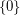# Invariant subspace problem

 Importance: High ✭✭✭
 Author(s):
 Subject: Analysis
 Keywords: subspace
 Posted by: tchow on: February 9th, 2009
Problem   Does every bounded linear operator on an infinite-dimensional separable Hilbert space have a non-trivial closed invariant subspace?

Letbe a Hilbert space. The subspacesandare trivially invariant under any linear operator on, and so these are referred to as the trivial invariant subspaces. The problem is concerned with determining whether bounded operators necessarily have non-trivial invariant subspaces.

This is one of the most famous open problems in functional analysis. Enflo  constructed Banach spaces for which the corresponding question has a negative answer, and recently Argyros and Haydon constructed a Banach space for which the corresponding question has a positive answer .

For a nice overview to the problem see ,  or .

## Bibliography

 P. Enflo, On the invariant subspace problem for Banach spaces, Acta Math. 158 (1987), 213-313. MathSciNet

 B. S. Yadav, The Present State and Heritages of the Invariant Subspace Problem, Milan J. Math. 73 (2005), 289-316. MathSciNet another link

 H. Radjavi and P. Rosenthal, The Invariant Subspace Problem, The Mathematics Intelligencer 4 (1982), no. 1, 33-37. MathSciNet

 S. A. Argyros and R. G. Haydon, A hereditarily indecomposable-space that solves the scalar-plus-compact problem, arXiv:0903.3921 (2009).

 J. Noel. The Invariant Subspace Problem. Honours Thesis, Thompson Rivers University. Link to pdf.

* indicates original appearance(s) of problem.

### closed

Does every bounded linear operator on an infinite-dimensional separable Hilbert space have a non-trivial closed invariant subspace?

### ستار اكاديمي 8

ستار اكاديمي 8

thank you man, u'r good :)